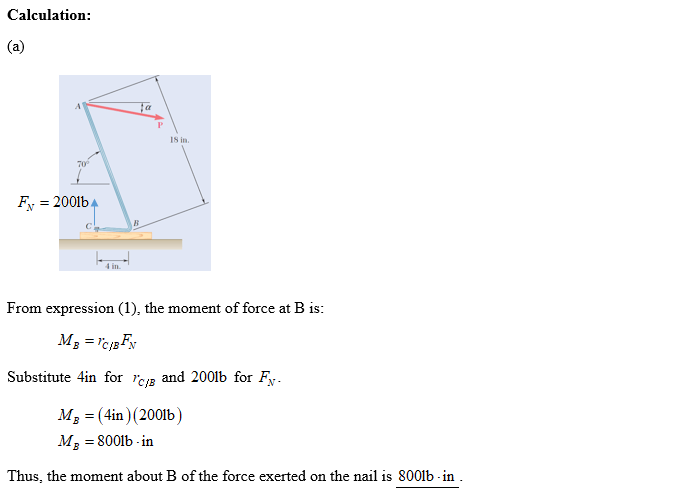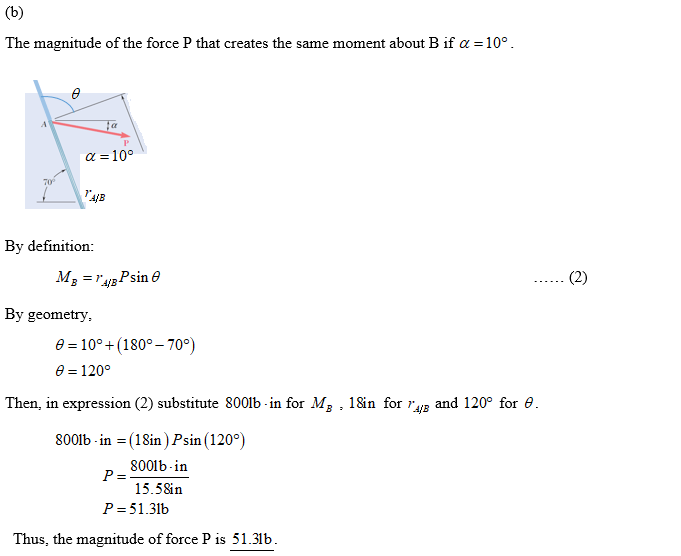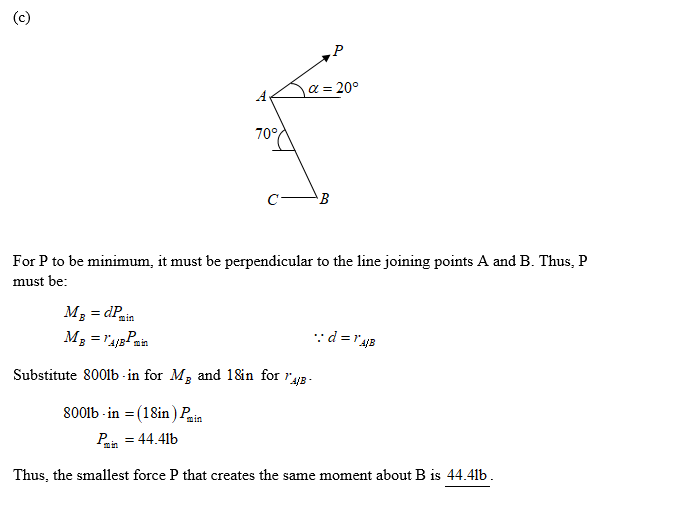# It is known that a vertical force of 200 lb is required to remove the nail at C from the board. As the nail first starts moving, determine (a) the moment about B of the force exerted on the nail, (b) the magnitude of the force P that creates the same moment about B if a = 10°, and (c ) the smallest force P that creates the same moment about B.

Question-AnswerCategory: Engineering MechanicsIt is known that a vertical force of 200 lb is required to remove the nail at C from the board. As the nail first starts moving, determine (a) the moment about B of the force exerted on the nail, (b) the magnitude of the force P that creates the same moment about B if a = 10°, and (c ) the smallest force P that creates the same moment about B.

It is known that a vertical force of 200 lb is required to remove the nail at C from the board. As the nail first starts moving, determine (a) the moment about B of the force exerted on the nail, (b) the magnitude of the force P that creates the same moment about B if a = 10°, and (c ) the smallest force P that creates the same moment about B.Step 1Step 2Step 3Step 4